Tue, Oct 04, 2022 @ 22:33 GMT
HomeArticlesTrading StrategiesExponential Moving Average Revealed

# Exponential Moving Average Revealed

A Moving Average is one of the most widely used indicators in the financial markets.

Like most indicators, it is very easy to apply on the price chart – you simply drag it onto the prices. Its interpretation is also fairly straightforward; buy above or sell below the moving average line. It’s no wonder that so many technical traders, even fundamentalists, use it for their analysis and trading.

Of course, moving averages come in many ‘flavours’. Simple Moving Average, Linear Weighted, Geometric, Exponential, Triangular, Volume Adjusted and Variable, to name a few.

If you are new to moving averages, you may be wondering which method is best.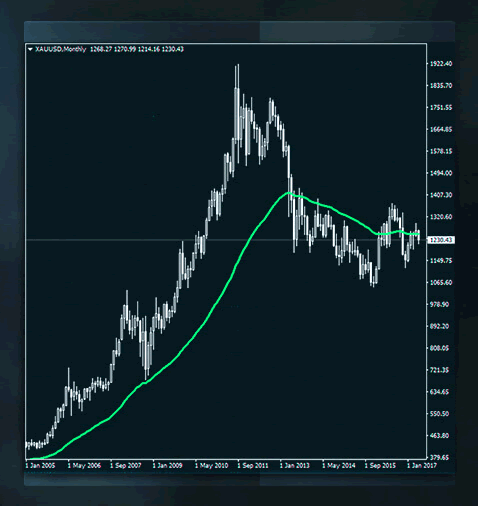Simple Moving Average (Arithmetic)

Most traders start experimenting with the Simple Moving Average (Arithmetic) in the early stages of their trading career.

This is calculated by adding the closing price of a number of candlesticks (equal to the time period of the moving average) and then dividing this number by the total number of prices. The result is known as the average. The oldest price is then dropped (i.e. discarded from the calculation) and the same formula is applied to the next prices. Therefore, it becomes the moving average.

For example, the calculation of a 5-period simple moving average begins with the prices from index 7 to 3, then the price of index 7 is dropped and the next average includes prices of index 6 to 2, after which the price of index 6 is discarded and the next calculation uses prices of index 5 to 1:

Index Price Average
7 1.10110
6 1.10120
5 1.10125
4 1.10140
3 1.10143 1.101276
2 1.10149 1.101354
1 1.10156 1.101426

Here is the formula in the form of a mathematical notation:

Simple Moving Average = [Price(n) + Price(n-1) + Price(n-2) + … + Price(1)] / n

Where n is the period of the moving average.

However, when investors gain more trading experience, they start to understand the disadvantages of the simple moving average. Lagging, for example, takes into account only those prices in the time interval and assigns equal weight to all prices in the period. In an attempt to address these types of issues, technical analysts came up with other calculation methods, such as the Exponential Moving Average (EMA).

EMA

This method addresses the biases inherent in the simple moving average, specifically issues with equal weight, since it only accounts for the prices in the predefined period. As a matter of fact, EMA takes into account all available prices in its calculation and assigns more weight to the most recent prices. Lagging, though, remains a disadvantage.

Here is the EMA formula:

EMA = (Price(i)*P)+(EMA(i-1)*(1-P))

Where P is the period and i is the candlestick index.

This is a recursive formula that even the most experienced traders would have difficulty understanding. Perhaps, if we write the formula in a different way, it will be easier to understand. For example:

EMA = (Price current – EMA previous) * multiplier + EMA previous

Where multiplier = 2/Periods + 1

Let’s take a look at the calculation of a 10-period Exponential Moving Average. Note that the oldest EMA (i.e. for index 10) is calculated as a Simple Moving Average of the previous prices.

Multiplier = 2/(Period+1)

= 2(10+1)

= 2/11

= 0.18182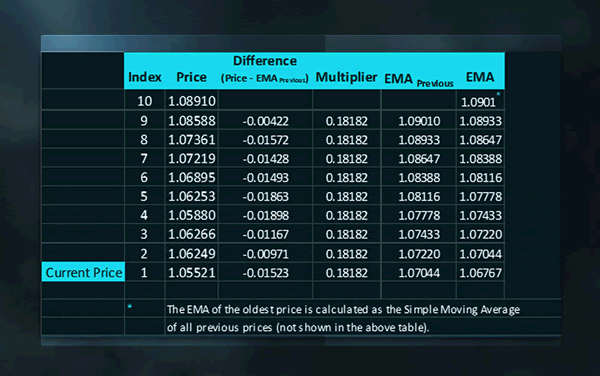Number of Periods

One of the biggest dilemmas a trader faces is deciding how many periods to use with the EMA.

There are a few variables to consider when deciding on the periodicity of a moving average, these include:

• Market Convention
• Constant
• Volatility
• Optimisation
• The predominant cycle

In the stock market, we notice that traders usually rely on moving averages that are 200 periods long. In the foreign exchange market, the period is much shorter; at times it’s 50, 20 or 10. Another decisive factor may be the volatility in the specific market. Trending markets imply shorter periods, where low volatility suggests longer lengths to avoid whipsaws (false signals). Frequent optimisation is another way of identifying the "best" possible period. On the other hand, some traders use a constant period for all markets and all timeframes. The choice is yours!

Trend Analysis

Trend identification is one of the easiest ways to use a moving average. When prices rise above the line, then an upward movement could be in the making.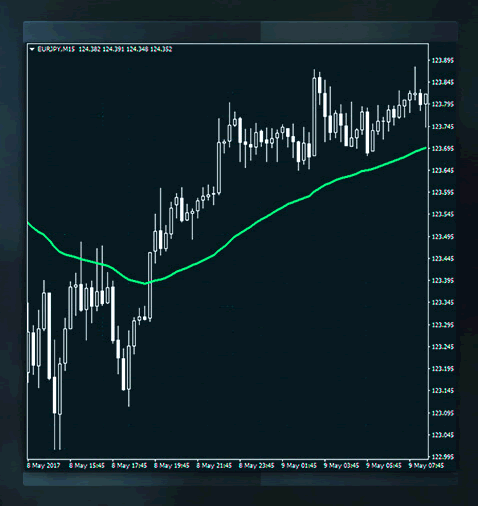On the other hand, when prices fall below the line, then a downward movement could be starting.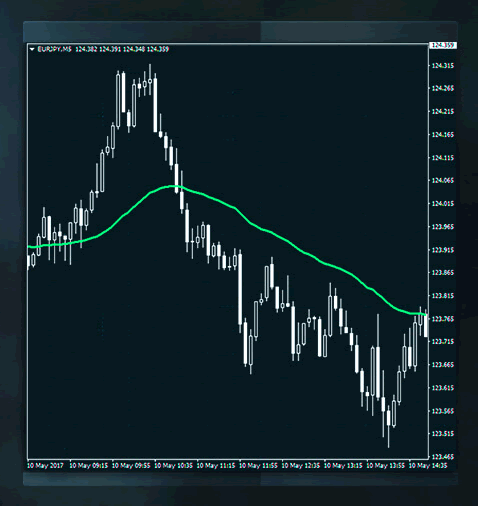Moving averages make extreme identification look very easy. When prices are far above the moving average, this may signal overbought trading. When prices are far below the moving average, it’s an indication that oversold levels may have been reached.

Interpretation

Just like the Simple Moving Average, a buy signal is generated when prices move above the moving average. Similarly, a sell signal is generated when prices fall below the moving average. To avoid false signals, price confirmation is needed most of the time. For example, a buy signal would require a close above the moving average line. Similarly, a close below would be needed for a sell signal.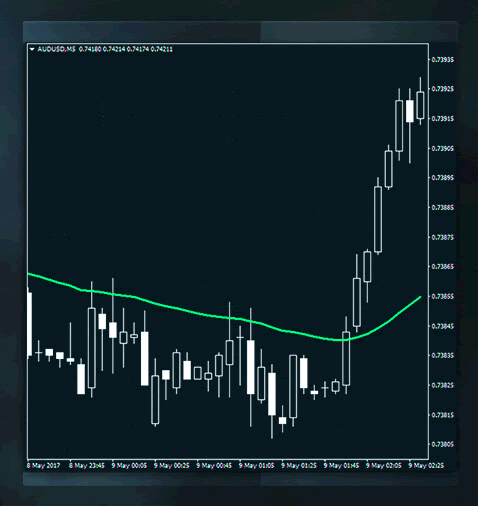Undoubtedly, whipsaws or false signals remain one of the major disadvantages of moving averages. One way to reduce them, is by waiting for the ‘tip’ of the moving average line to turn upwards before placing a buy order, or to point downwards when placing a short position. Needless to say, all signals require confirmation by the price itself.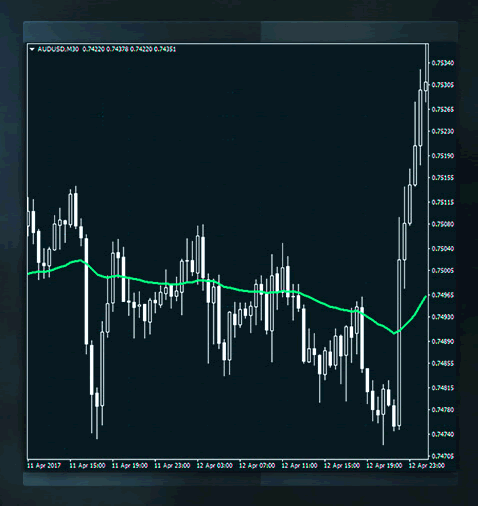Of course, moving averages may be combined with other indicators and oscillators to provide traders with greater precision.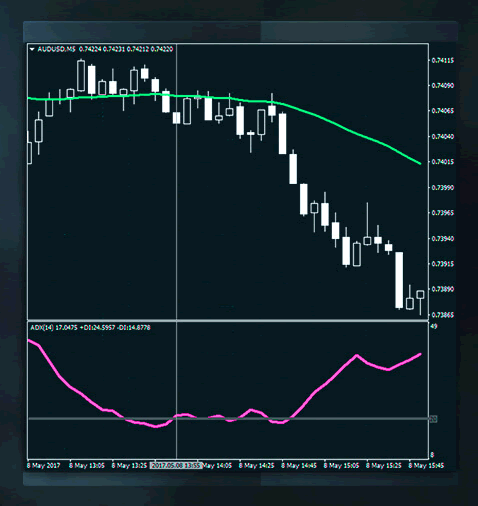Conclusion

The Exponential Moving Average is a useful tool that is part of many traders’ arsenals. Its simplicity in trend identification, as well as the fact that this method addresses both criticisms of the simple moving average, makes it the method of choice for many traders. Unfortunately, combined with the delay in signals, false signals can cause difficulties when caught in a sideways market.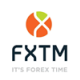ForexTimehttp://www.forextime.com/
The FXTM brand provides international brokerage services and gives access to the global currency markets, offering trading in forex, precious metals, Share CFDs, ETF CFDs and CFDs on Commodity Futures. Trading is available via the MT4 and MT5 platforms with spreads starting from just 1.3 on Standard trading accounts and from 0.1 on ECN trading accounts. Bespoke trading support and services are provided based on each client's needs and ambitions - from novices, to experienced traders and institutional investors. ForexTime Limited is regulated by the Cyprus Securities and Exchange Commission (CySEC), with license number 185/12, licensed by South Africa's FSB with FSP number 46614, and registered with the UK FCA under reference number 600475. FT Global Limited is regulated by the International Financial Services Commission (IFSC) with license numbers IFSC/60/345/TS and IFSC/60/345/APM.